# 坑 7 文献

## 7.1 文献管理

### 7.1.3 从精到用

• 我的研究究竟有没有实际意义？
• 我的发现是否有助于学科发展或写入教科书？
• 我现在纠结的事10年20年后会不会纠结？

## 7.2 文本挖掘

### 7.2.1 关键词

# remotes::install_github("yufree/scifetch")
library(scifetch)
library(lubridate)
##
## Attaching package: 'lubridate'
## The following objects are masked from 'package:base':
##
##     date, intersect, setdiff, union
library(dplyr)
##
## Attaching package: 'dplyr'
## The following objects are masked from 'package:stats':
##
##     filter, lag
## The following objects are masked from 'package:base':
##
##     intersect, setdiff, setequal, union
library(tidytext)
library(stringr)
library(ggplot2)
## Keep up to date with changes at
## https://www.tidyverse.org/blog/
library(tidyr)
library(broom)
library(purrr)
library(scales)
##
## Attaching package: 'scales'
## The following object is masked from 'package:purrr':
##
##     discard
# 10年跨度 MeSH 关键词 期刊ES&T
query <- '"mass spectrometry"[MeSH Terms] AND 2010/01:2020/01[DP] AND 0013-936X[TA]'
tmdf <- getpubmed(query, start = 1, end = 10000) %>%
getpubmedtbl() %>%
mutate(time = as.POSIXct(date, origin = "1970-01-01"),
month = round_date(date, "month"))
## 822 records founds
# 摘要分词
wordfabs <- tmdf %>%
filter(nchar(abstract) > 0) %>%
unnest_tokens(word, abstract,drop = F) %>%
anti_join(stop_words) %>%
filter(str_detect(word, "[^\\d]")) %>%
filter(!str_detect(word, "abs")) %>%
group_by(word) %>%
mutate(word_total = n()) %>%
ungroup() %>%
mutate(source = 'abstract')
## Joining, by = "word"
# 可视化词频前20的关键词
wordfabs %>%
count(word, sort = TRUE) %>%
top_n(20,n) %>%
mutate(word = reorder(word, n)) %>%
ggplot(aes(word, n)) +
geom_col(show.legend = FALSE) +
ylab("Top 20 commonly used words in abstracts") +
coord_flip()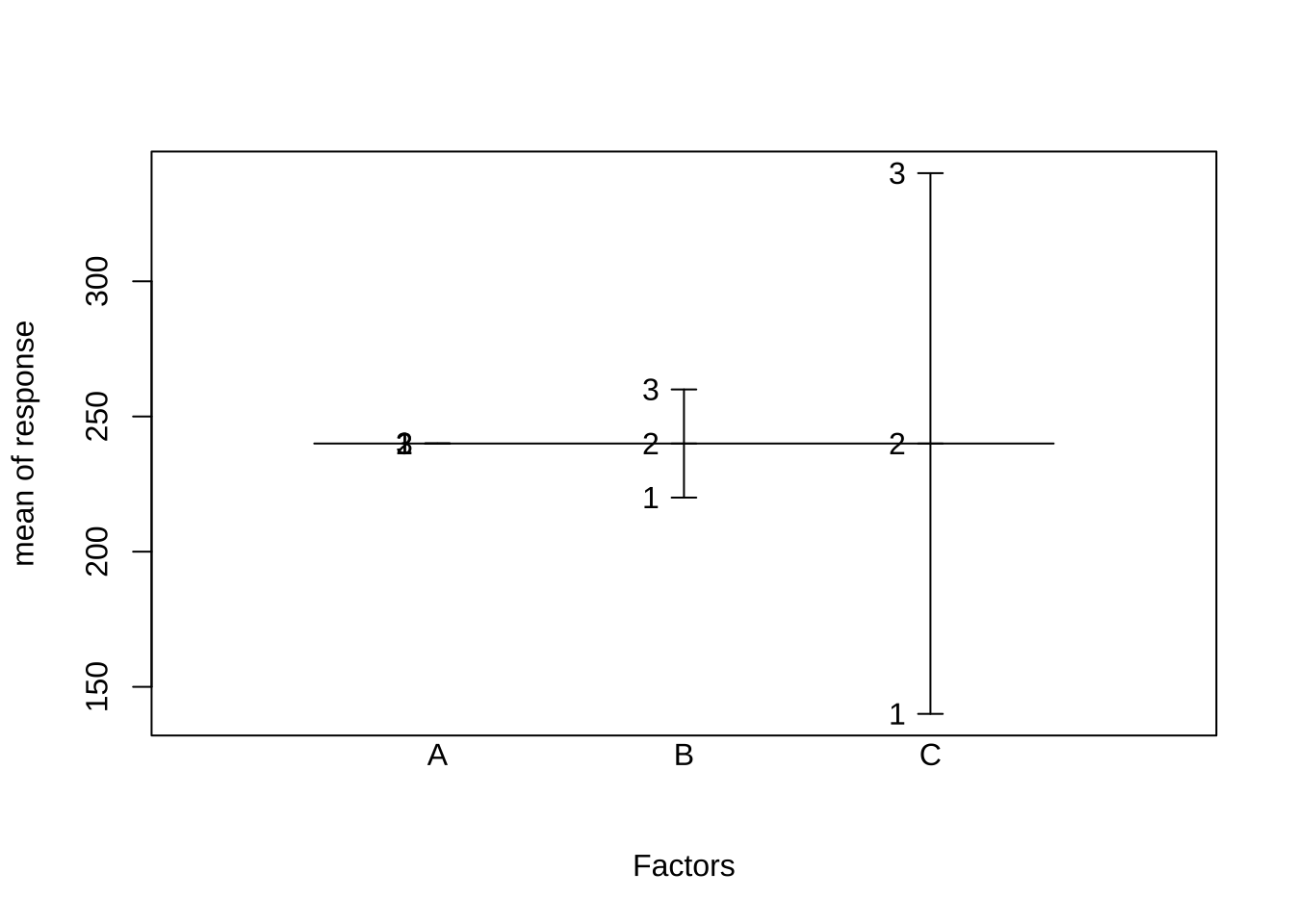papers_per_month <- tmdf %>%
group_by(month) %>%
summarize(month_total = n())
# 摘要中关键词
word_month_counts <- wordfabs %>%
filter(word_total >= 100) %>%
count(word, month) %>%
complete(word, month, fill = list(n = 0)) %>%
inner_join(papers_per_month, by = "month") %>%
mutate(percent = n / month_total) %>%
mutate(year = year(month) + yday(month) / 365) %>%
filter(percent<1)
# 计数数据广义二项回归
mod <- ~ glm(cbind(n, month_total - n) ~ year, ., family = "binomial")
# 计算斜率
slopes <- word_month_counts %>%
nest(-word) %>%
mutate(model = map(data, mod)) %>%
mutate(models = map(model,tidy)) %>%
unnest(cols = c(models)) %>%
filter(term == "year") %>%
arrange(desc(estimate))
## Warning: All elements of ... must be named.
## Did you want data = c(month, n, month_total, percent, year)?
# 快速成长关键词
slopes %>%
head(9) %>%
inner_join(word_month_counts, by = "word") %>%
mutate(word = reorder(word, -estimate)) %>%
ggplot(aes(month, n / month_total, color = word)) +
geom_line(show.legend = FALSE) +
scale_y_continuous(labels = percent_format()) +
facet_wrap(~ word, scales = "free_y") +
expand_limits(y = 0) +
labs(x = "Year",
y = "Percentage of abstracts containing this term",
title = "9 fastest growing words",
subtitle = "Judged by growth rate"
)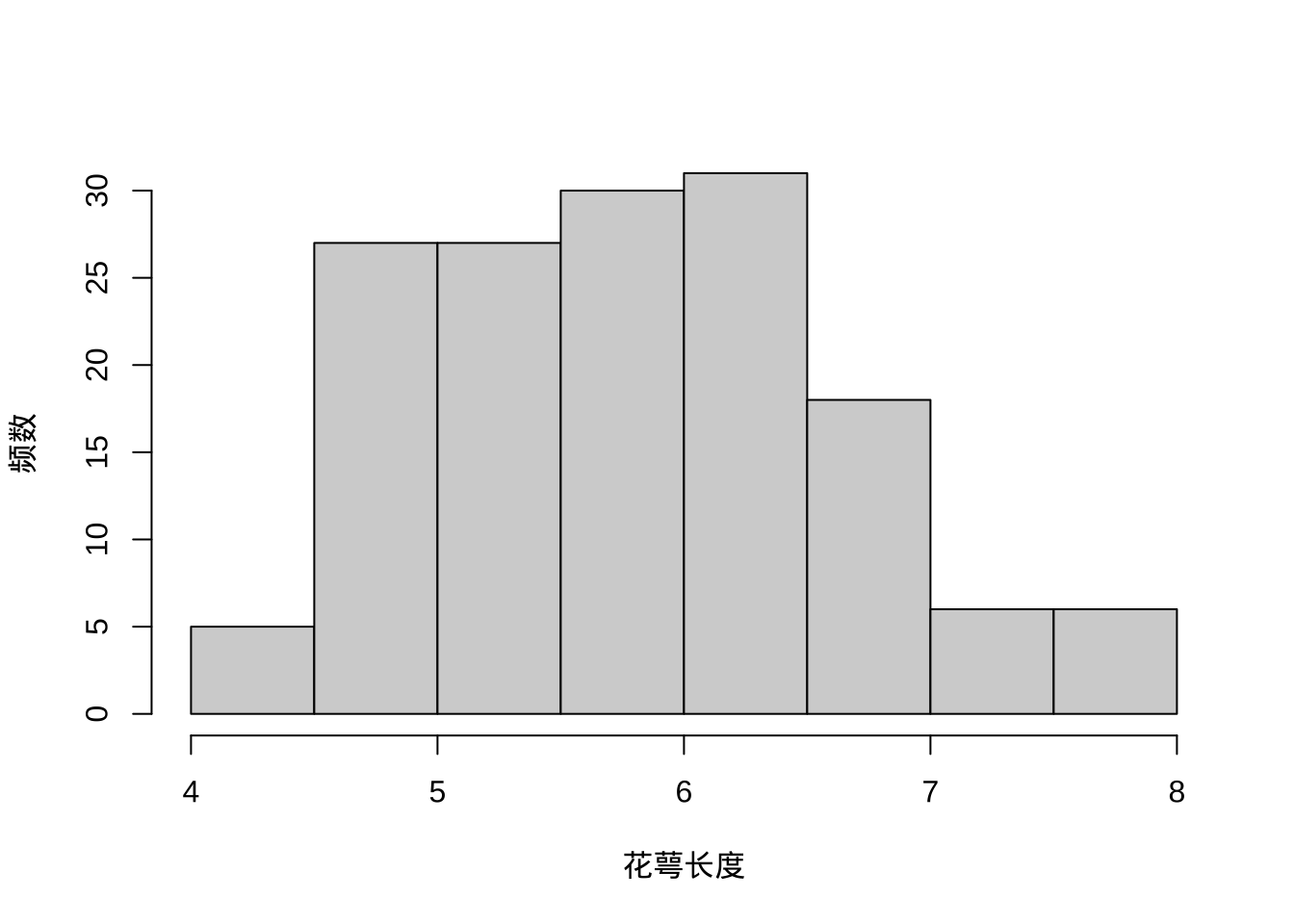# 衰退关键词
slopes %>%
tail(9) %>%
inner_join(word_month_counts, by = "word") %>%
mutate(word = reorder(word, estimate)) %>%
ggplot(aes(month, n / month_total, color = word)) +
geom_line(show.legend = FALSE) +
scale_y_continuous(labels = percent_format()) +
facet_wrap(~ word, scales = "free_y") +
expand_limits(y = 0) +
labs(x = "Year",
y = "Percentage of abstracts containing this term",
title = "9 fastest shrinking words",
subtitle = "Judged by growth rate"
)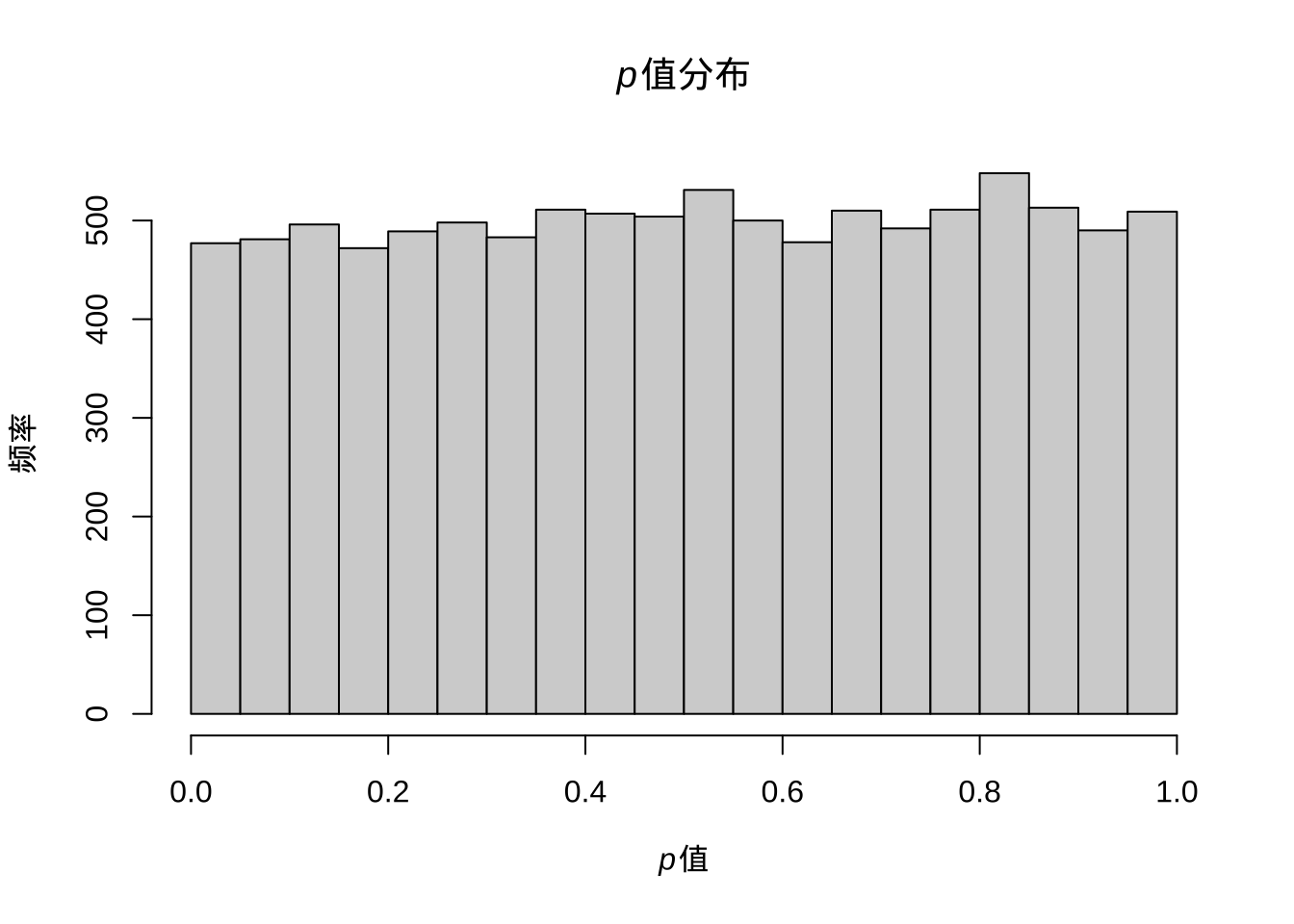library(widyr)
library(igraph)
##
## Attaching package: 'igraph'
## The following objects are masked from 'package:purrr':
##
##     compose, simplify
## The following object is masked from 'package:tidyr':
##
##     crossing
## The following objects are masked from 'package:dplyr':
##
##     as_data_frame, groups, union
## The following objects are masked from 'package:lubridate':
##
##     %--%, union
## The following objects are masked from 'package:stats':
##
##     decompose, spectrum
## The following object is masked from 'package:base':
##
##     union
library(ggraph)

title_word_pairs <- wordfabs %>%
pairwise_count(word,line,sort = TRUE)
## Warning: distinct_() was deprecated in dplyr 0.7.0.
## Please use distinct() instead.
## See vignette('programming') for more help
## Warning: tbl_df() was deprecated in dplyr 1.0.0.
## Please use tibble::as_tibble() instead.
set.seed(42)
title_word_pairs %>%
filter(n >= 150) %>%
graph_from_data_frame() %>%
ggraph(layout = "fr") +
geom_edge_link(aes(edge_alpha = n, edge_width = n), edge_colour = "cyan4") +
geom_node_point(size = 1) +
geom_node_text(aes(label = name), repel = TRUE,
point.padding = unit(0.2, "lines")) +
labs(title = "Bigrams in title") +
theme_void()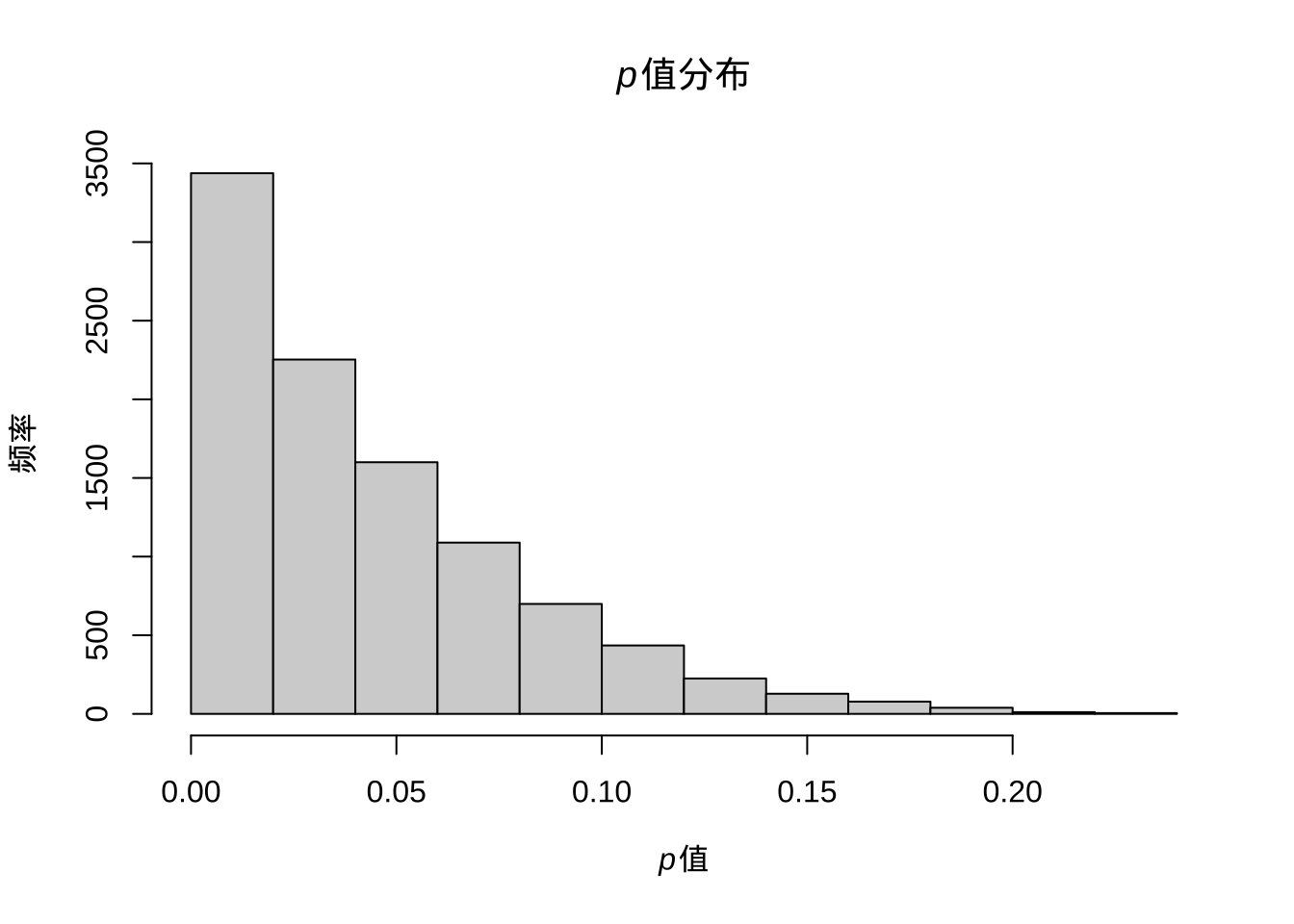desc_dtm <- wordfabs %>%
count(line, word, sort = TRUE) %>%
ungroup() %>%
cast_dtm(line, word, n)
library(topicmodels)
desc_lda <- LDA(desc_dtm, k = 6, control = list(seed = 42))
tidy_lda <- tidy(desc_lda)

top_terms <- tidy_lda %>%
group_by(topic) %>%
top_n(10, beta) %>%
ungroup() %>%
arrange(topic, -beta)

top_terms %>%
mutate(term = reorder(term, beta)) %>%
group_by(topic, term) %>%
arrange(desc(beta)) %>%
ungroup() %>%
mutate(term = factor(paste(term, topic, sep = "__"),
levels = rev(paste(term, topic, sep = "__")))) %>%
ggplot(aes(term, beta, fill = as.factor(topic))) +
geom_col(show.legend = FALSE) +
coord_flip() +
scale_x_discrete(labels = function(x) gsub("__.+$", "", x)) + labs(title = "Top 10 terms in each LDA topic", x = NULL, y = expression(beta)) + facet_wrap(~ topic, ncol = 2, scales = "free")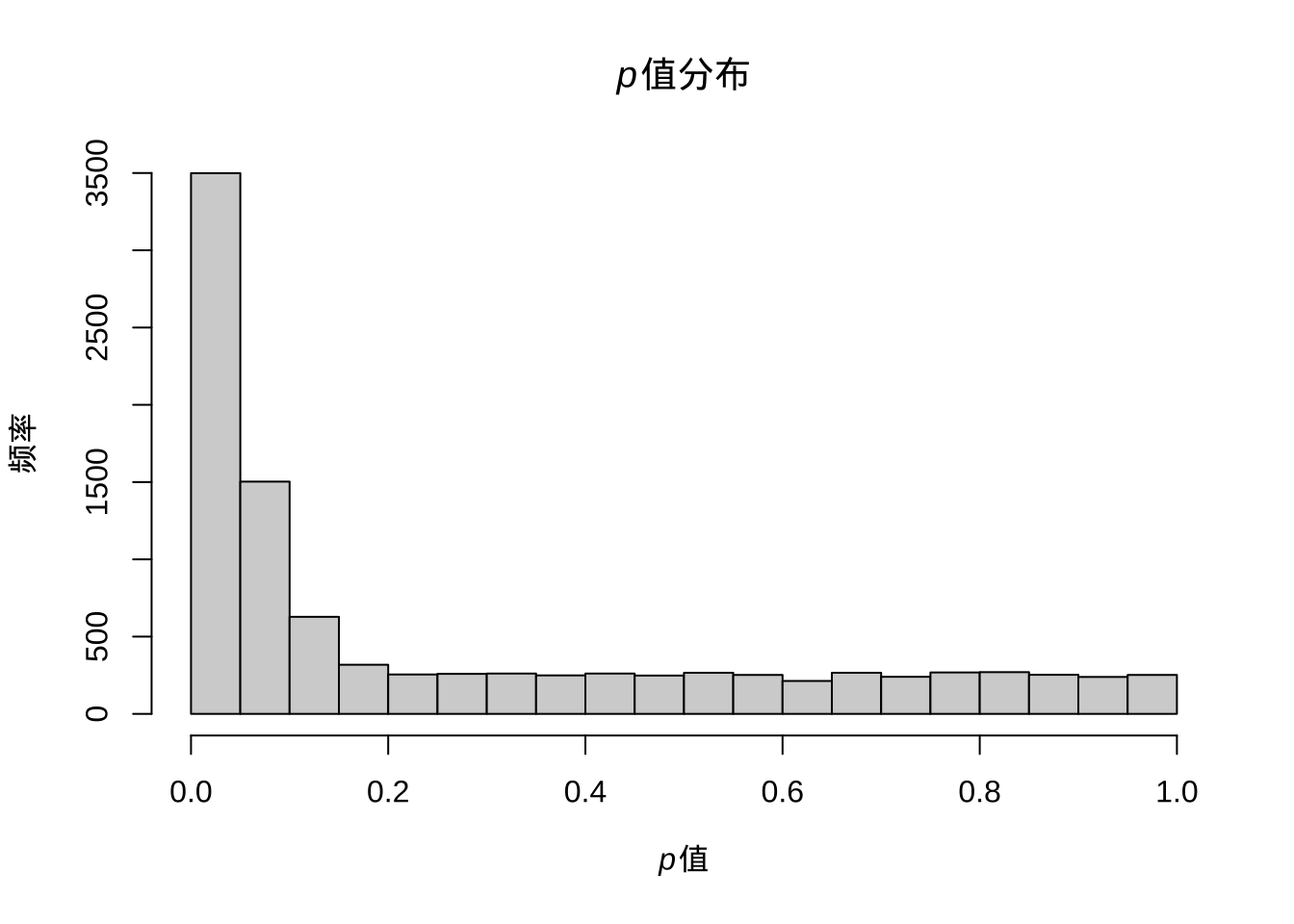主题模型会尝试将相关性高的词归为一个主题，这里我们大体可以推测出第一个主题是关于气相色谱物质鉴定的；第二个主题是关于水中天然溶解性有机物分析；第三个大概是水样灰尘样中污染物定量研究；第四个大概是土壤降解转化产物；第五个是气溶胶反应产物；第六个则是水样的分析方法研究。这样，通过文本挖掘我们就可以推测出一些通常需要阅读很多文献才能形成的学科发展趋势。 ### 7.2.2 作者 同样的，我们也可以研究某个作者的研究主题与变化趋势。这里用加拿大滑铁卢大学 Jaunsz Pawliszyn 教授的数据来说明下。 # 10年跨度 query <- 'janusz pawliszyn[AU] AND 2010/01:2020/01[DP]' tmdf <- getpubmed(query, start = 1, end = 10000) %>% getpubmedtbl() %>% mutate(time = as.POSIXct(date, origin = "1970-01-01"), month = round_date(date, "month")) ## 195 records founds # 摘要分词 wordfabs <- tmdf %>% filter(nchar(abstract) > 0) %>% unnest_tokens(word, abstract,drop = F) %>% anti_join(stop_words) %>% filter(str_detect(word, "[^\\d]")) %>% filter(!str_detect(word, "abs")) %>% group_by(word) %>% mutate(word_total = n()) %>% ungroup() %>% mutate(source = 'abstract') ## Joining, by = "word" papers_per_month <- tmdf %>% group_by(month) %>% summarize(month_total = n()) # 可视化词频前20的关键词 wordfabs %>% count(word, sort = TRUE) %>% top_n(20,n) %>% mutate(word = reorder(word, n)) %>% ggplot(aes(word, n)) + geom_col(show.legend = FALSE) + ylab("Top 20 commonly used words in abstracts") + coord_flip()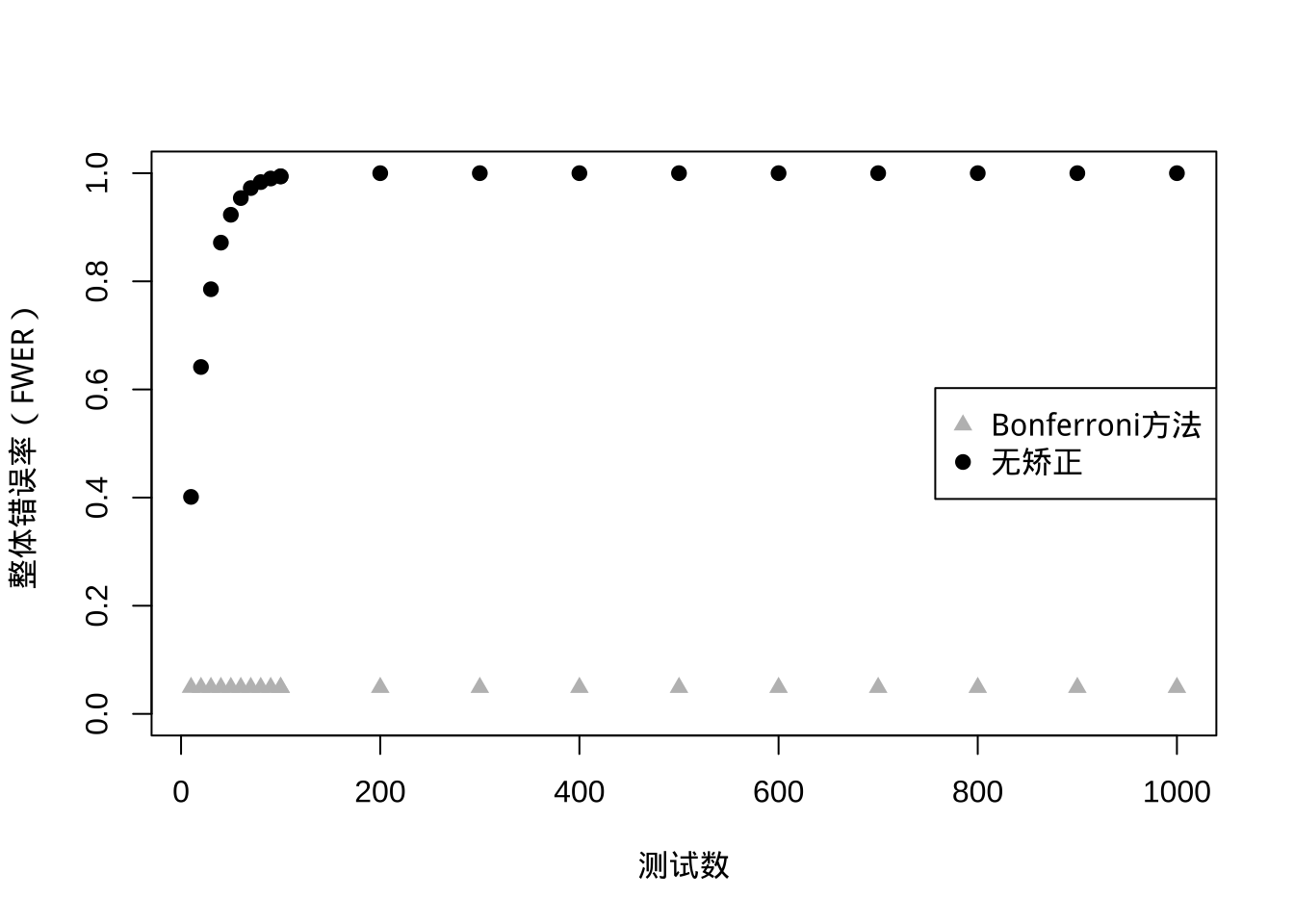# 摘要中关键词 word_month_counts <- wordfabs %>% filter(word_total >= 100) %>% count(word, month) %>% complete(word, month, fill = list(n = 0)) %>% inner_join(papers_per_month, by = "month") %>% mutate(percent = n / month_total) %>% mutate(year = year(month) + yday(month) / 365) %>% filter(percent<1) # 计数数据广义二项回归 mod <- ~ glm(cbind(n, month_total - n) ~ year, ., family = "binomial") # 计算斜率 slopes <- word_month_counts %>% nest(-word) %>% mutate(model = map(data, mod)) %>% mutate(models = map(model,tidy)) %>% unnest(cols = c(models)) %>% filter(term == "year") %>% arrange(desc(estimate)) ## Warning: All elements of ... must be named. ## Did you want data = c(month, n, month_total, percent, year)? # 快速成长关键词 slopes %>% head(9) %>% inner_join(word_month_counts, by = "word") %>% mutate(word = reorder(word, -estimate)) %>% ggplot(aes(month, n / month_total, color = word)) + geom_line(show.legend = FALSE) + scale_y_continuous(labels = percent_format()) + facet_wrap(~ word, scales = "free_y") + expand_limits(y = 0) + labs(x = "Year", y = "Percentage of abstracts containing this term", title = "9 fastest growing words", subtitle = "Judged by growth rate" )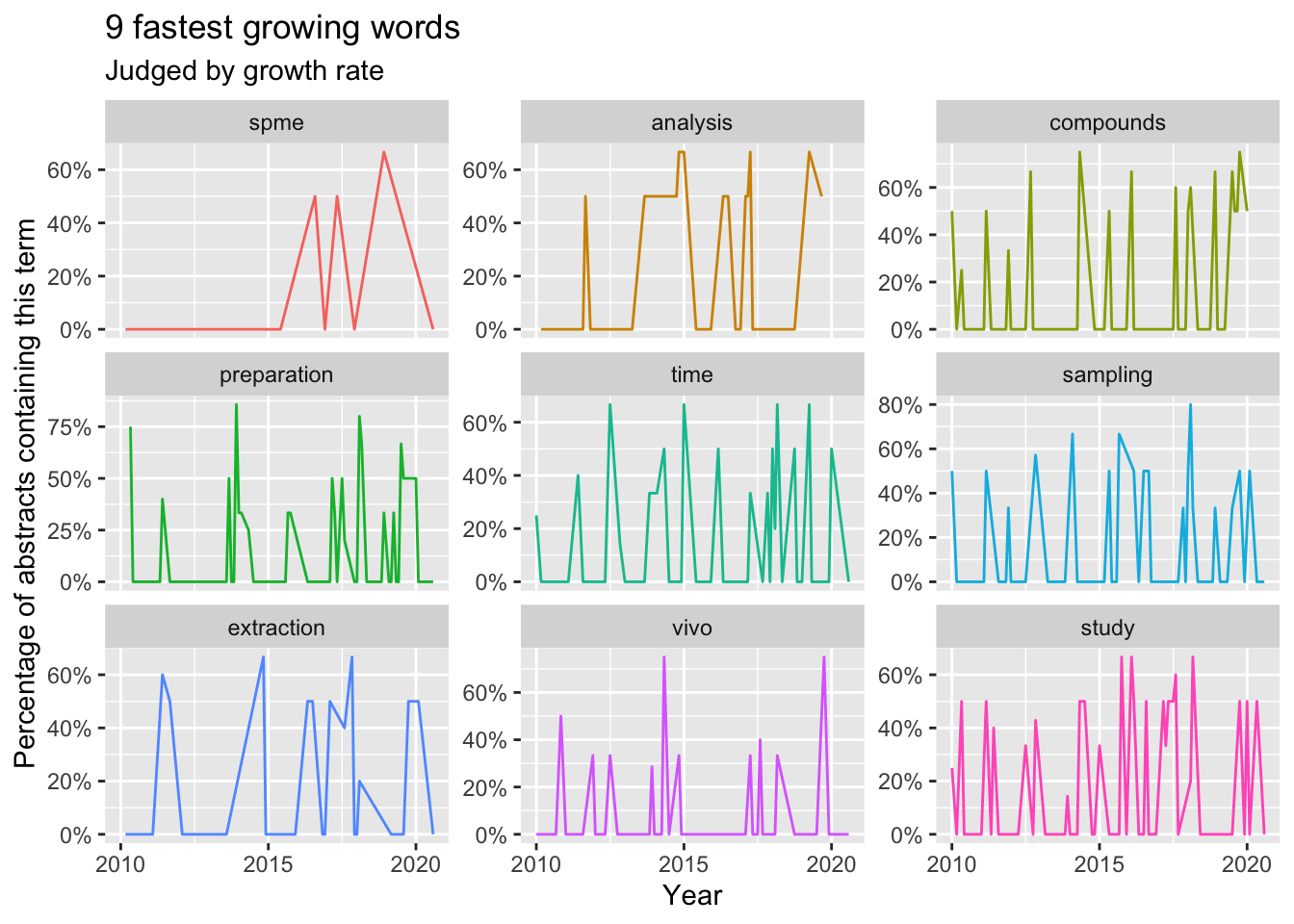# 衰退关键词 slopes %>% tail(9) %>% inner_join(word_month_counts, by = "word") %>% mutate(word = reorder(word, estimate)) %>% ggplot(aes(month, n / month_total, color = word)) + geom_line(show.legend = FALSE) + scale_y_continuous(labels = percent_format()) + facet_wrap(~ word, scales = "free_y") + expand_limits(y = 0) + labs(x = "Year", y = "Percentage of abstracts containing this term", title = "9 fastest shrinking words", subtitle = "Judged by growth rate" )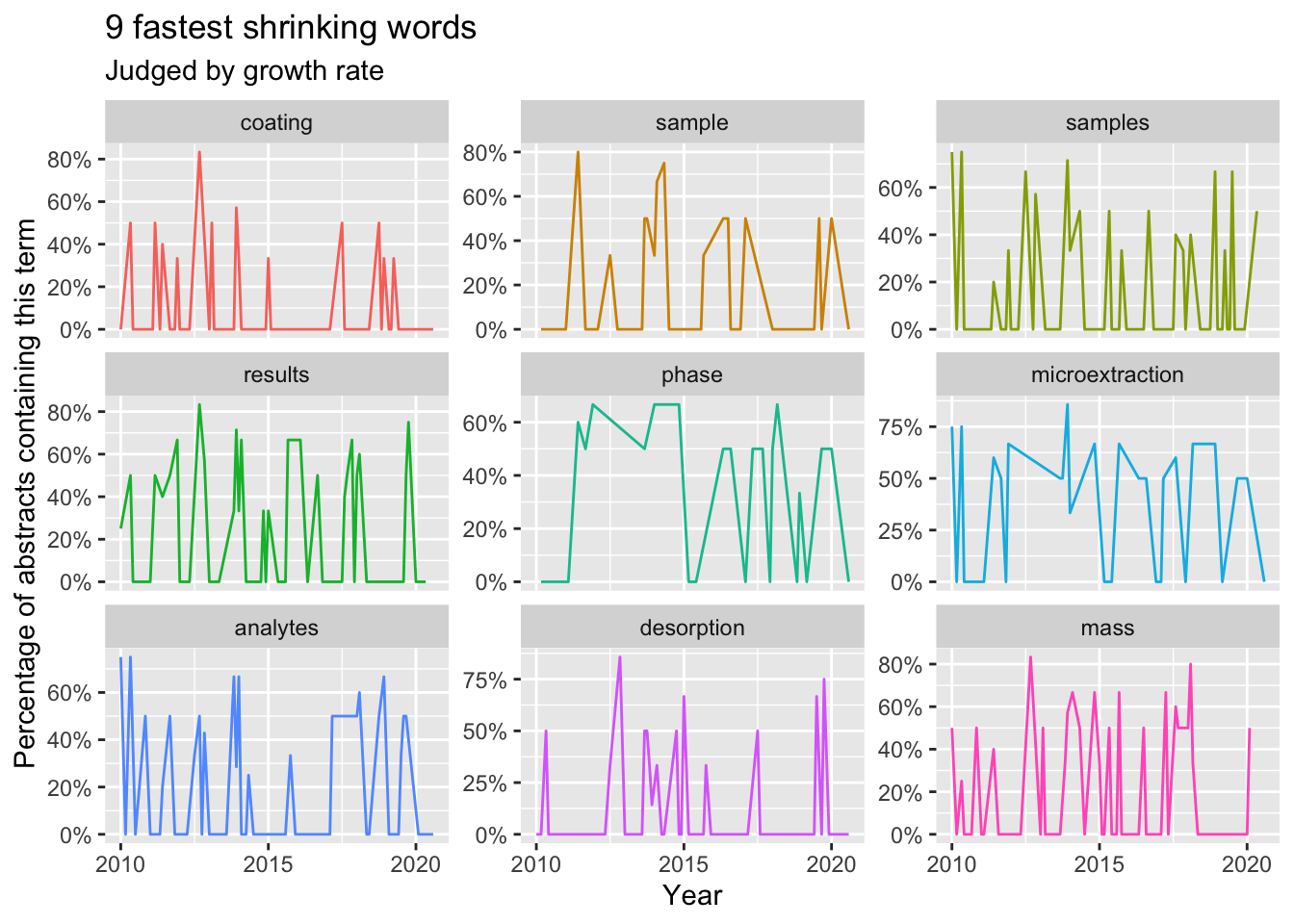同样，我们可以研究下其发表的期刊偏好。 table(tmdf$journal)[order(table(tmdf\$journal),decreasing = T)][1:10]   
##
##              Anal Chem         Anal Chim Acta
##                     44                     33
##         J Chromatogr A            Bioanalysis
##                     32                      9
##    Environ Sci Technol              J Sep Sci
##                      7                      7
## Angew Chem Int Ed Engl                Sci Rep
##                      6                      6
##                Talanta                Analyst
##                      6                      4

## 7.3 全学科评价

• S级 年同行发文量超过 5 万的关键词领域，疑似有停滞点
• A级 年同行发文量 1 万到 5 万的关键词领域，有停滞点
• B级 年同行发文量 2 千到 1 万的关键词领域，有停滞点
• C级 年同行发文量 100 到 2 千的关键词领域
• D级及以下 年同行发文量 100 以下的关键词领域

## 7.4 荟萃分析

$\hat\theta_F = \frac{\sum\limits_{k=1}^K \hat\theta_k/ \hat\sigma^2_k}{\sum\limits_{k=1}^K 1/\hat\sigma^2_k}$